Hello. Those who follow my blog know that lately I have started to share, in the form of articles, what I learn in my personal study of statistics.

In practice, I share my notes on this fascinating subject with you in order to help those who, like me, have embarked on a personal study journey, and also to be able to correct and "adjust the aim" if a concept is misunderstood.

In my last article, I talked about what I believe are the three main branches of statistics: descriptive statistics, probability theory, and inferential statistics. Now it's time to delve into them by dedicating one or more articles to each of them.

In this article, we will begin to discuss descriptive statistics in detail, so get yourself a nice cup of tea, sit back comfortably, and enjoy reading.

Imagine being hired by a large agricultural company. Your boss wants to test a product recommended by a consultant who claims it can increase the number of berries produced by each tomato plant it is applied to. Of course, your boss is interested in verifying if this is true. They have no intention of spending a bunch of money on a product that doesn't bring any benefit to production. They ask you to determine whether this product is actually capable of increasing berry production.

You have several options to answer your boss. For example, you could apply the product to one tomato plant and count the number of berries produced after a certain number of days, comparing them with the berry production of an untreated plant. But that wouldn't make sense. Who assures us that the increased berry production in the treated plant is actually due to the applied product? The difference in production could be due to chance or other factors and not to the applied product. It is clear that this first strategy doesn't make sense because you need to test the product on multiple plants and compare them with an equal or very similar number of untreated plants.

So, you decide to test the product on 20 tomato plants and compare the berry production with another 20 untreated tomato plants. And now? Well, you need to collect the data systematically.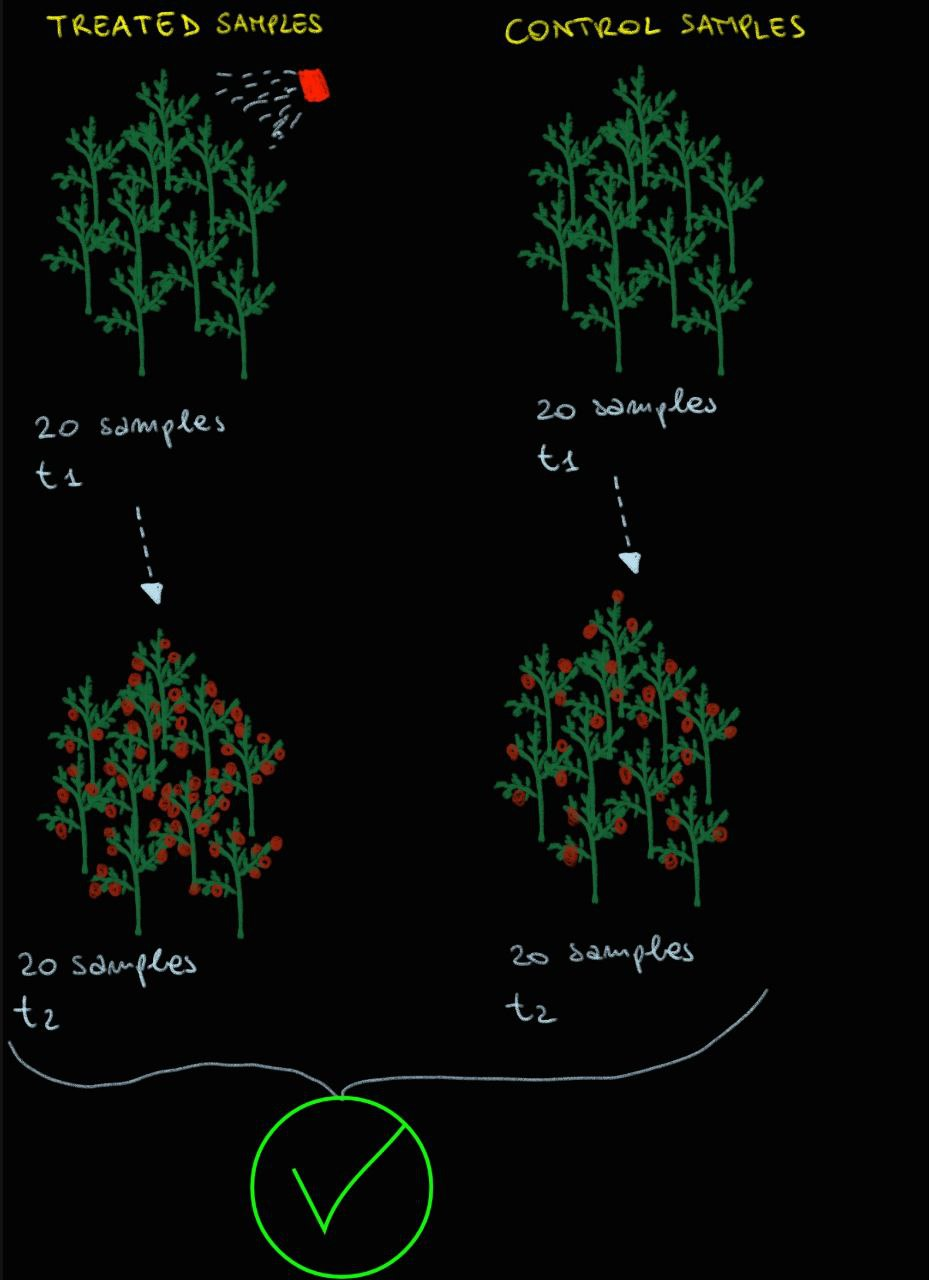Create a table with three columns and 41 rows (20 for the treated plants + 20 for the untreated plants + the first row with the variable names). The first column contains an identification number for each plant (see image below), the second column contains the values of the "Treatment Status" variable, and the third column contains the values of the "number of berries produced" variable corresponding to each plant.At this point, you have the tools to describe the data and tell your boss if there are differences between the treated plants and the control plants in terms of the berries produced. It's quite intuitive that presenting the entire table with the different values of berries produced per plant doesn't make much sense. Your boss is a busy person and wants a quick and easy-to-understand answer. Therefore, we need to summarize the collected values.

To summarize the available data, we can use summary indices, which are values that individually provide important information about the data under examination.

In this regard, descriptive statistics can help us. Within descriptive statistics, there are two important types of summary indices: central tendency or position indices and variability indices.Measures of central tendency provide information about the values of the variables under examination, giving us a quick idea of their magnitudes.

There are several measures of central tendency, such as:

• Mean
• Median
• Mode
• Minimum and maximum values
• Quantiles

The mean is a measure used to summarize the values of quantitative variables and can take different forms. The arithmetic mean is generally the most well-known and commonly used. The weighted mean is used when we have data with different weights, where some data points are more important than others, and this importance is usually indicated by another variable. For example, university professors in Italy use the weighted mean. A grade of 30 obtained in an exam with a weight of 12 credits (representing the variable used to describe the weights) is certainly more valuable than a grade of 30 obtained in an exam worth 4 credits. Therefore, when calculating a student's average grade, this weighting should be taken into account. There is also the harmonic mean, which is used when the data in question are ratios. For example, the average speed of our vehicle is a ratio, expressed in km/h, which is distance divided by time. Finally, there is the geometric mean, particularly useful for summarizing values that vary over time. For example, the geometric mean is used to calculate the average annual weight gain of our pet.

Of course, each type of mean is calculated using a mathematical formula, as you can see from the summary diagram below: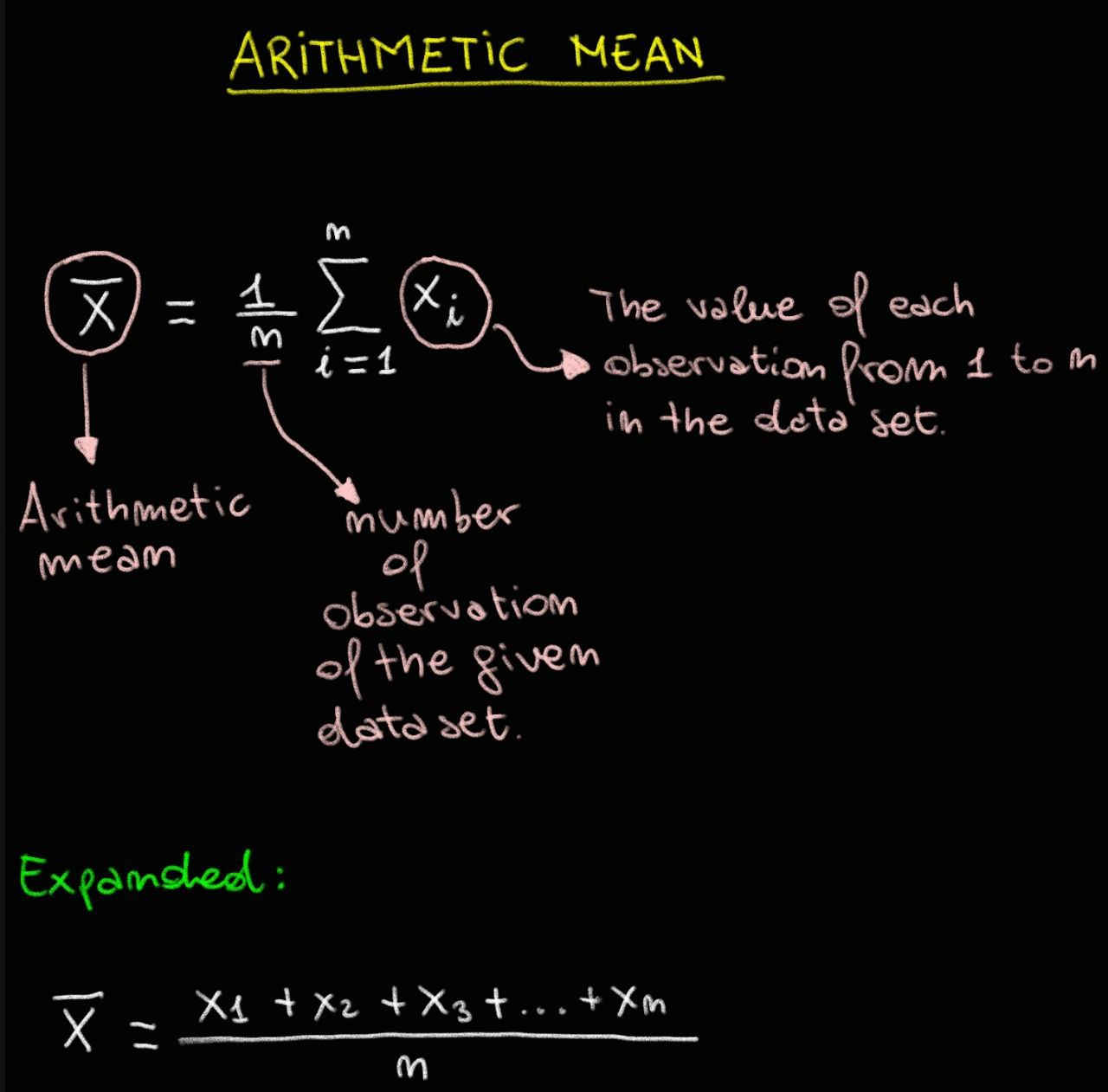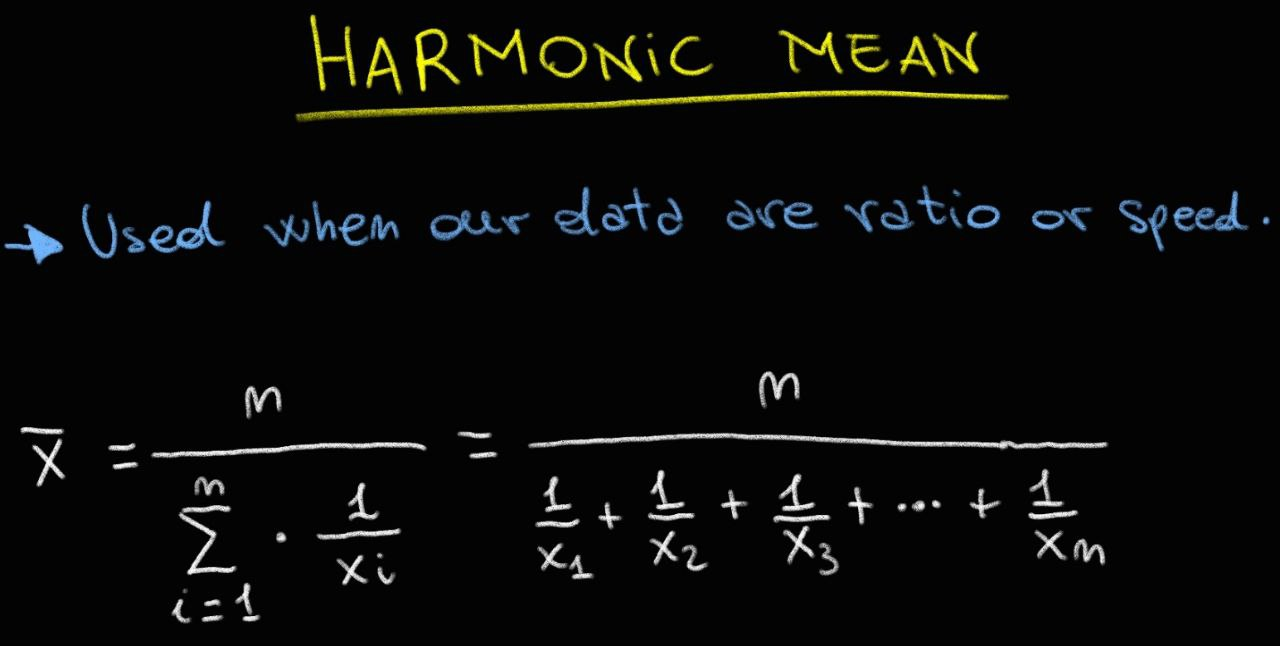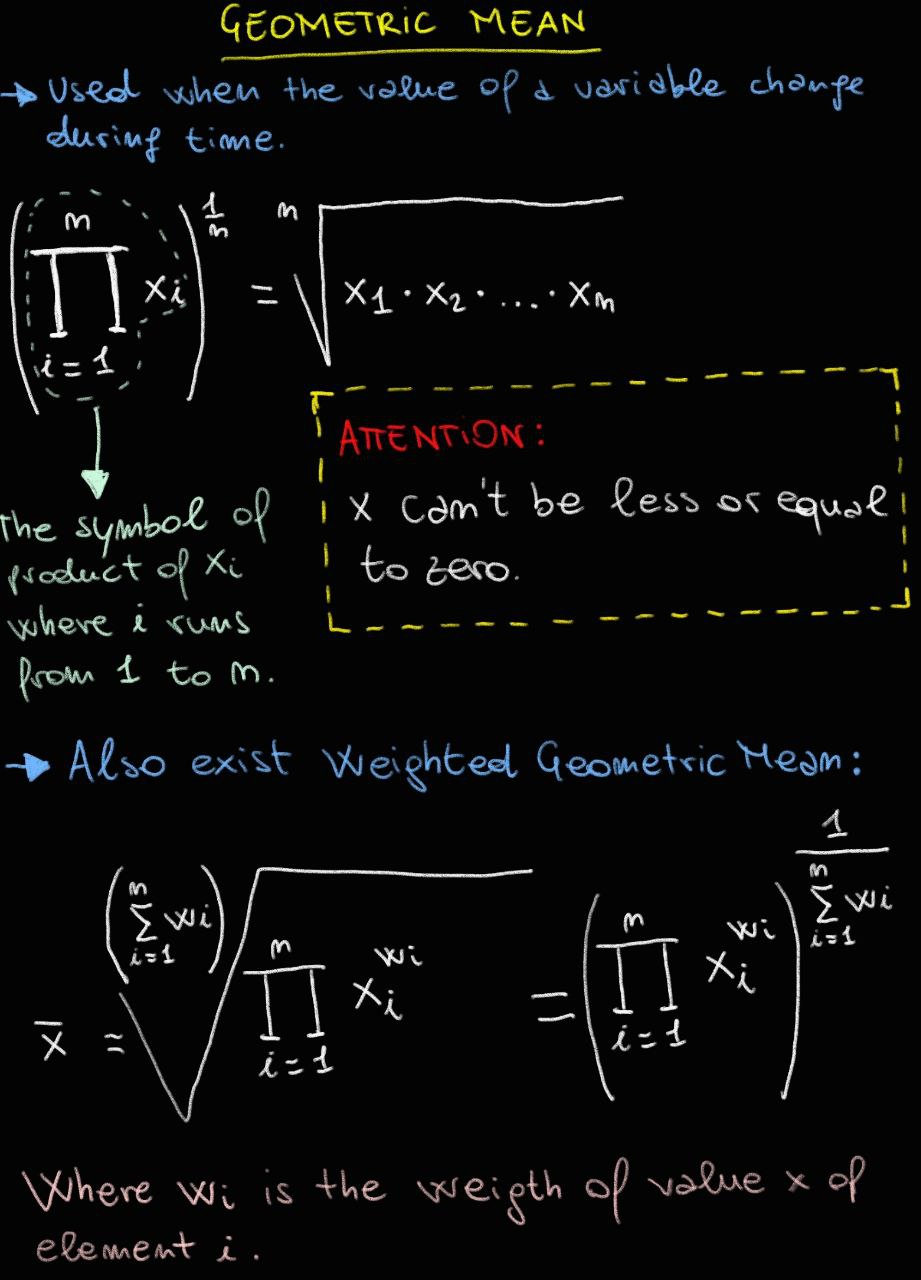The median is a very interesting measure. Arrange your data in ascending or descending order, lined up like soldiers. The median corresponds to the value that is exactly in the middle of your data set.In the case of data grouped into classes, as you can see in the example below, the "median class" is defined as the first class that has a cumulative relative frequency greater than or equal to 0.5 (i.e., 50%).There is a useful consideration we can make when comparing the mean and the median. It is known that the median is less influenced by extreme values, called "outliers," compared to the mean, which is more affected by them. Therefore, when there are many outliers, it can be useful to use the median instead of the mean.

The mode is a measure used to summarize the values of nominal qualitative variables (e.g., hair color). It corresponds to the most frequent value in the available dataset. For better understanding, you can refer to the example illustrated in the diagram below.The minimum and maximum values of a quantitative variable in the dataset under examination can be used as summary indices. These measures of central tendency allow us to identify the range and outliers in a data set.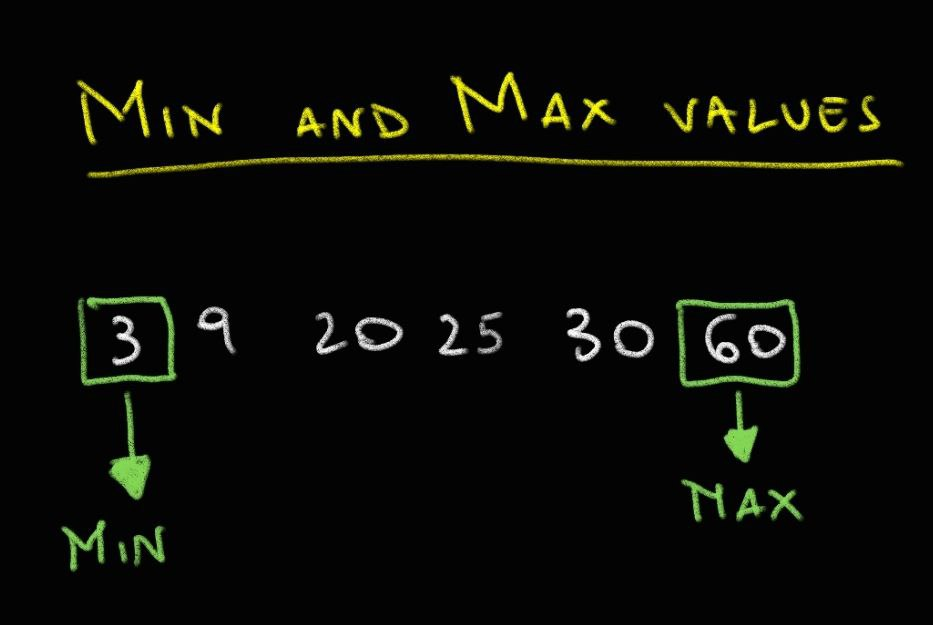Lastly, quantiles are numerical values that divide the dataset into equal parts. The median is technically a quantile since it divides the data into two equal parts. In fact, the median is considered the 50th quantile or 0.5. Other commonly used quantiles for dividing the data include the 25th quantile or 0.25, also known as the first quartile (Q1), which represents the value below which 25% of the data lies; and the 75th quantile or 0.75, also known as the third quartile (Q3), which represents the value below which 75% of the data lies.

Therefore, by definition, a quantile is a threshold value that divides the data into equal parts. If four thresholds are used to divide the data into four equal parts, they are called quartiles. Similarly, if you want to divide the data into one hundred equal parts, you would use one hundred thresholds, known as percentiles.

Feeling overwhelmed by all this? Don't worry, watch this video created by Joshua Starmer, PhD. Trust me, no one explains complex concepts better than him.

Well, your boss is waiting for your answer, remember? Which measure of central tendency can help us summarize the values of the variable "number of berries produced" in our dataset?

So, let's think calmly. The number of berries produced is a continuous quantitative variable. In this case, we could use the arithmetic mean to summarize the data.

Once we calculate the mean for the treated and untreated plants, we obtain an easily interpretable value that succinctly explains the behavior of the plants after the treatment. Of course, we could also calculate the median if we believe there are outliers that excessively influence the arithmetic mean.

Now we know that, on average, the treated plants produce 12 berries, while the untreated ones produce 6. It seems that the product recommended by the consultant delivers on its promise, but wait a moment. Rushing to your boss with this statement could be dangerous. In fact, central tendency indices have a significant limitation: they are unable to describe the variation in values within the available dataset. If I were to tell you that the mean of the berries produced in the treated plants is 8, but the values are distributed among the twenty plants as shown in the image below, with 10 plants not producing any berries, would you still go and tell your boss that the tested product is excellent? Certainly not. For a farmer, it's not a good sign to have plants that require resources but are not productive.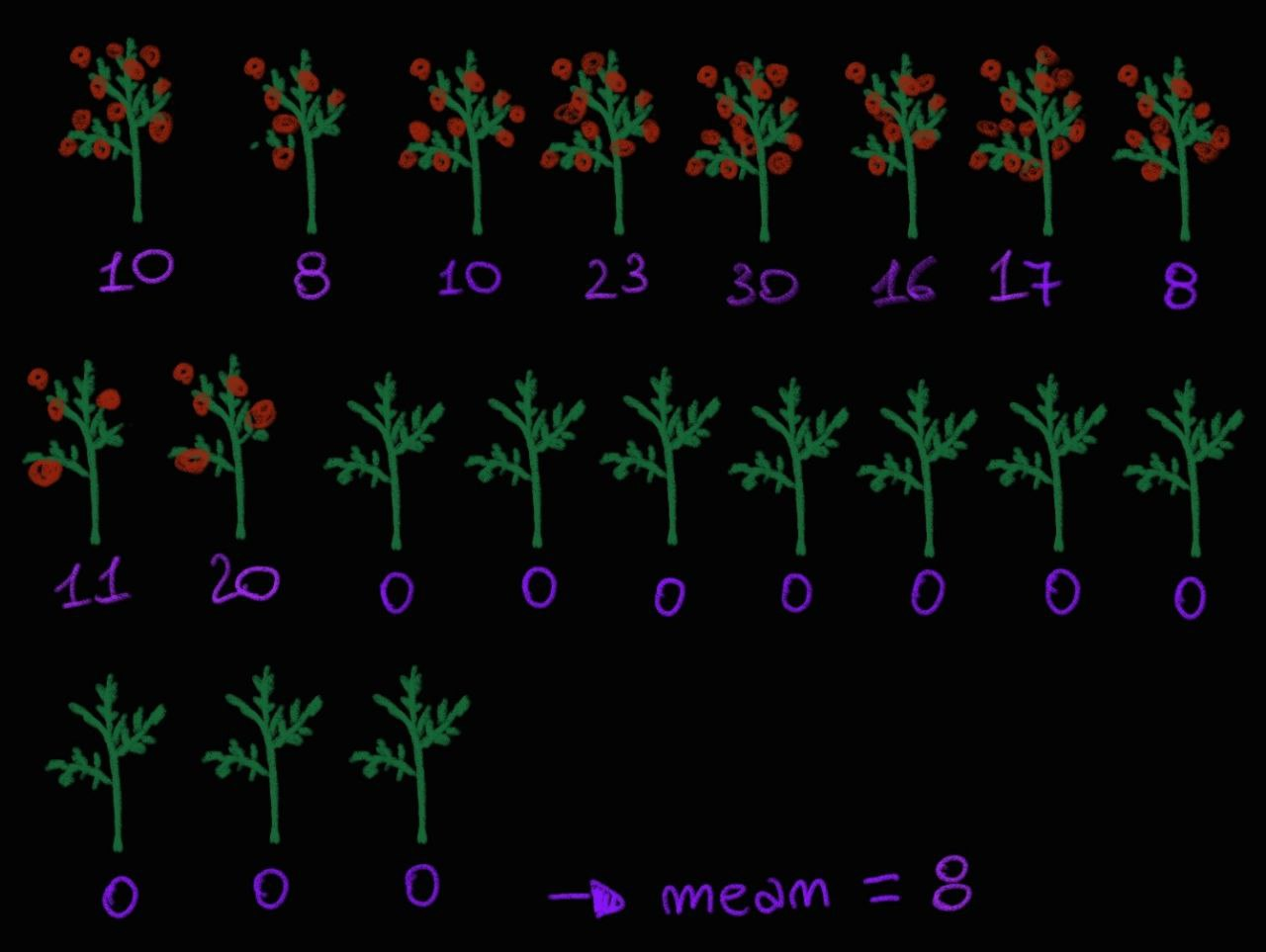So, we need to add a measure of variability, which is a numerical value that allows us to understand how much the values vary within the dataset.

There are different variability indices , including:

• Range or range of variation.
• Interquartile range.
• Variance.
• Standard deviation.
• Coefficient of variation.
• Gini coefficient of heterogeneity.

The range helps us understand within which interval the values in question oscillate. It corresponds to the difference between the maximum value and the minimum value in the dataset.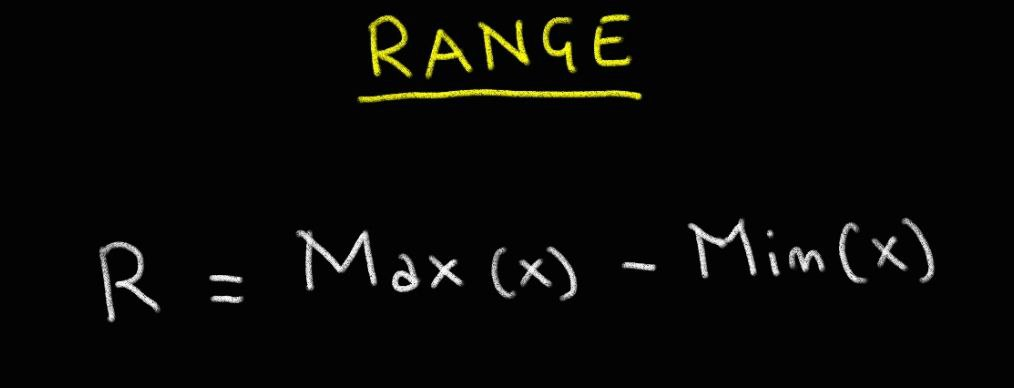The interquartile range is the difference between the third quartile (Q3) and the first quartile (Q1). This helps us understand the variation of the middle 50% of the data, as Q1 and Q3 represent the boundaries that "enclose" the 50% of values. The interquartile range is particularly useful when there are many outliers since this measure of variability is not influenced by their presence.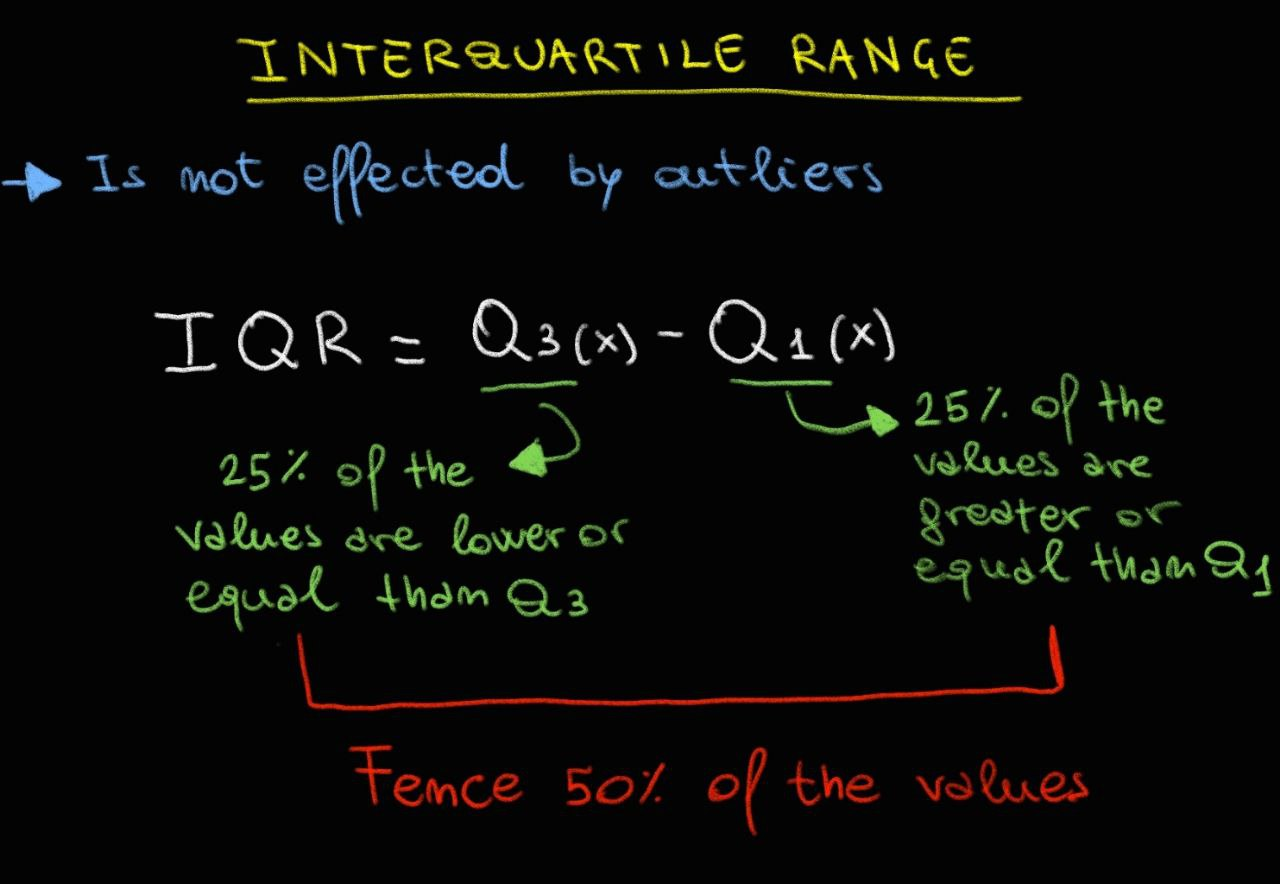Variance is a widely used measure of variability as it indicates the spread of data around the mean (which represents the central point of the value distribution). As can be easily understood from the example below, variance has a significant limitation as it tends to be on a different scale being a squared value. Therefore, it cannot be used to compare the variation of values of a variable between different datasets.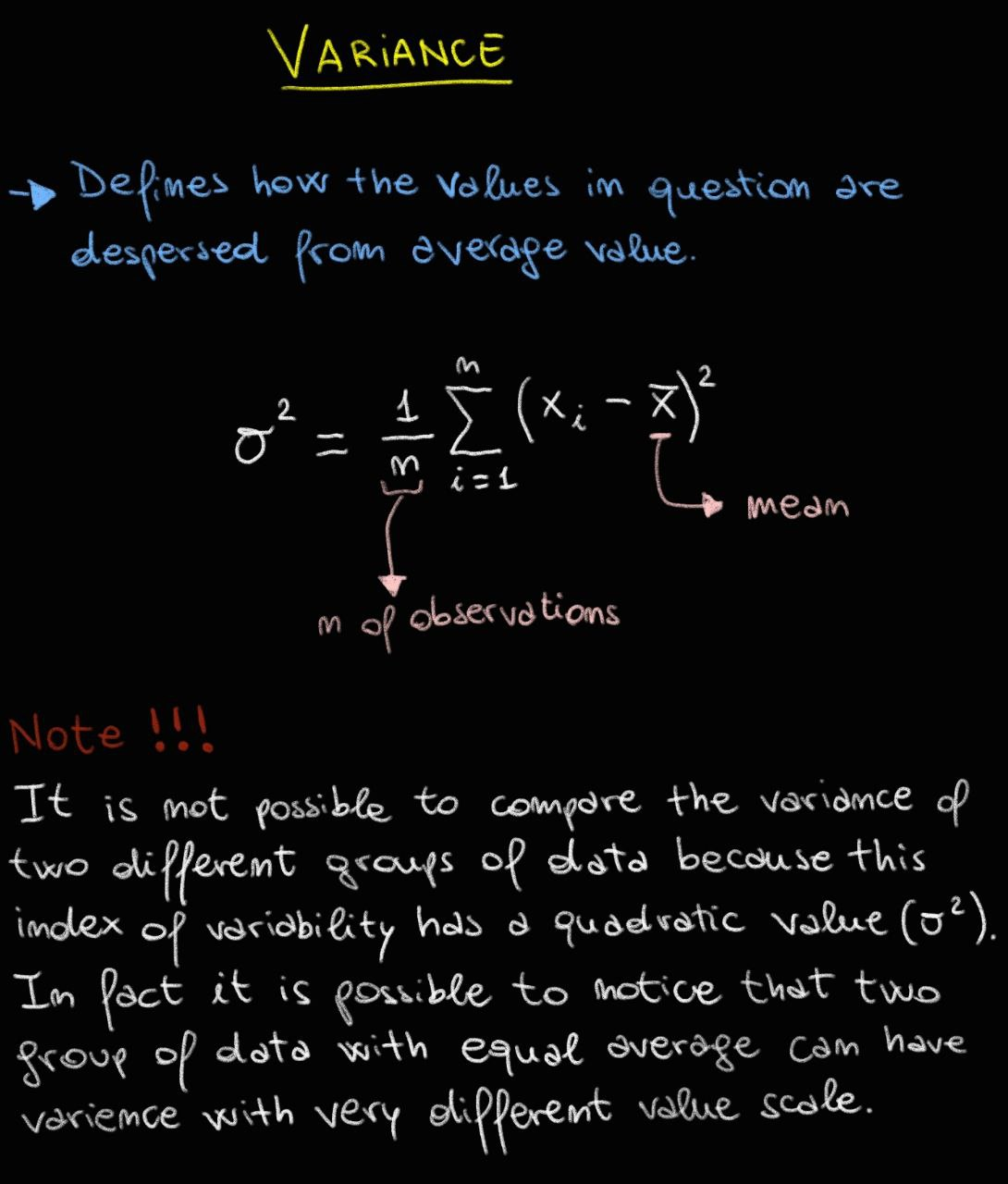Standard deviation is the measure that overcomes the limitations of variance, as it corresponds to the square root of variance. The square root allows for rescaling the variance values to make them comparable, as shown in the example below. However, caution must be exercised when using standard deviation to compare different data. If the mean values of the two datasets being compared are significantly different, the standard deviation is not suitable for comparing highly dissimilar datasets.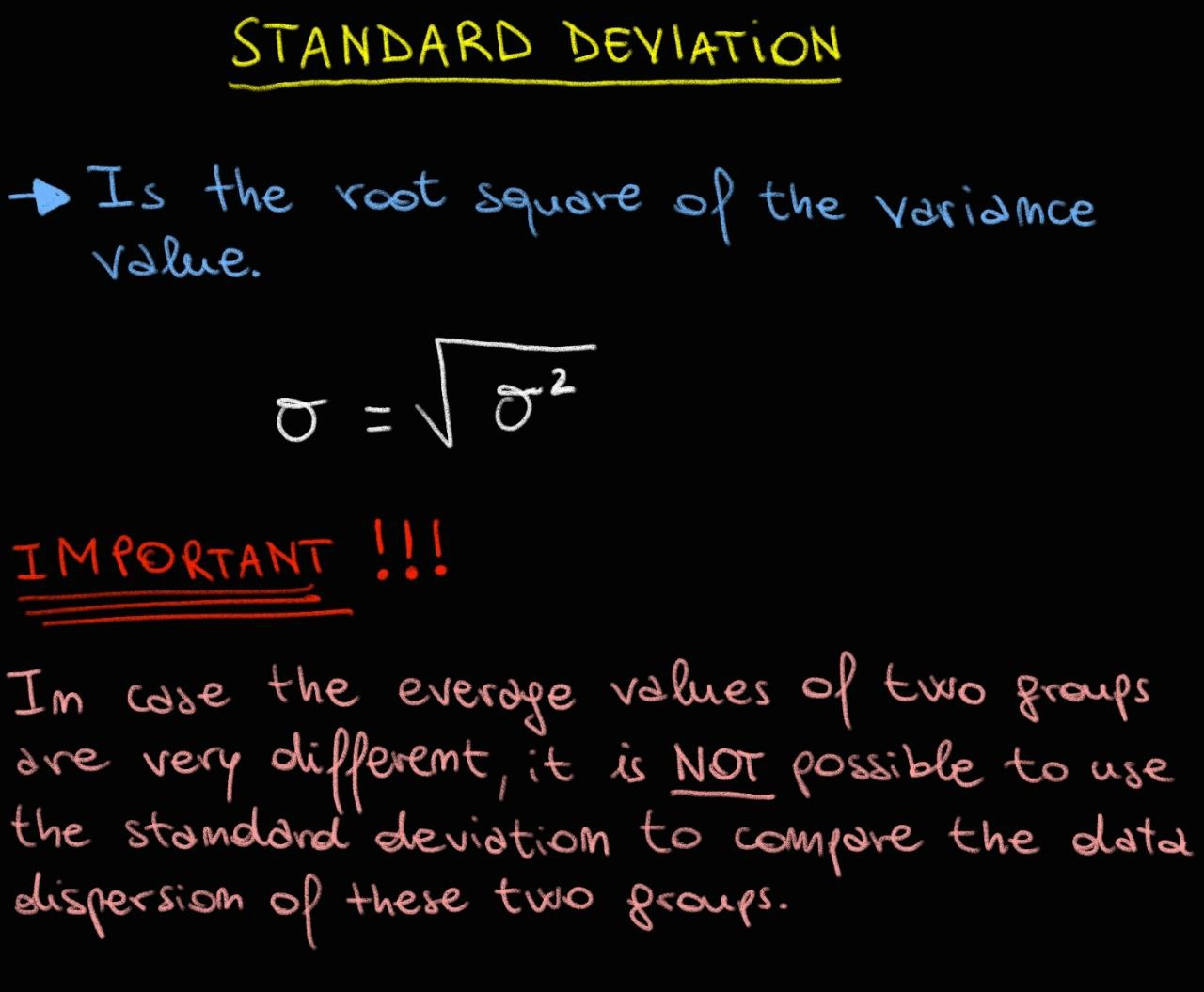The coefficient of variation is an extremely useful index because it allows us to:

• Compare two samples relative to the same variable.
• Compare a sample relative to two different variables.

The coefficient of variation is calculated by dividing the standard deviation of a variable by its mean and expressing the result as a percentage. This index provides a measure of relative variability, taking into account the magnitude of the variable's values. It allows for comparisons even when the means of the variables or samples being compared are different.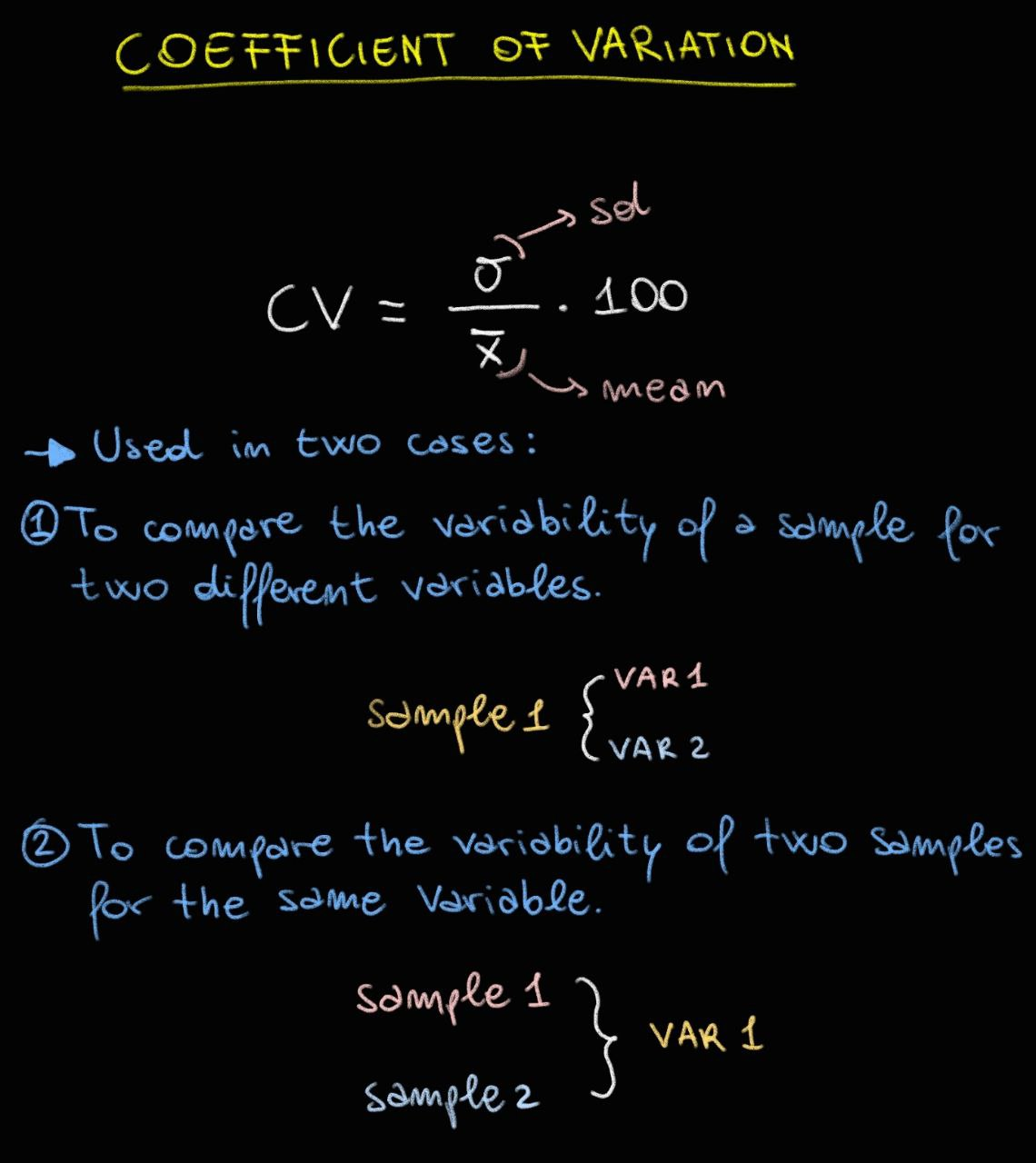Look at the image below to understand how we could use the variation coefficient as tool for comparison variability:Attention: Using the coefficient of variation becomes complicated when the variable under consideration has both positive and negative values.

Finally, there is the Gini heterogeneity index, which allows us to assess the variability of a qualitative variable. This index measures the tendency of a qualitative variable to take on its different values, considering the frequency distribution of those values.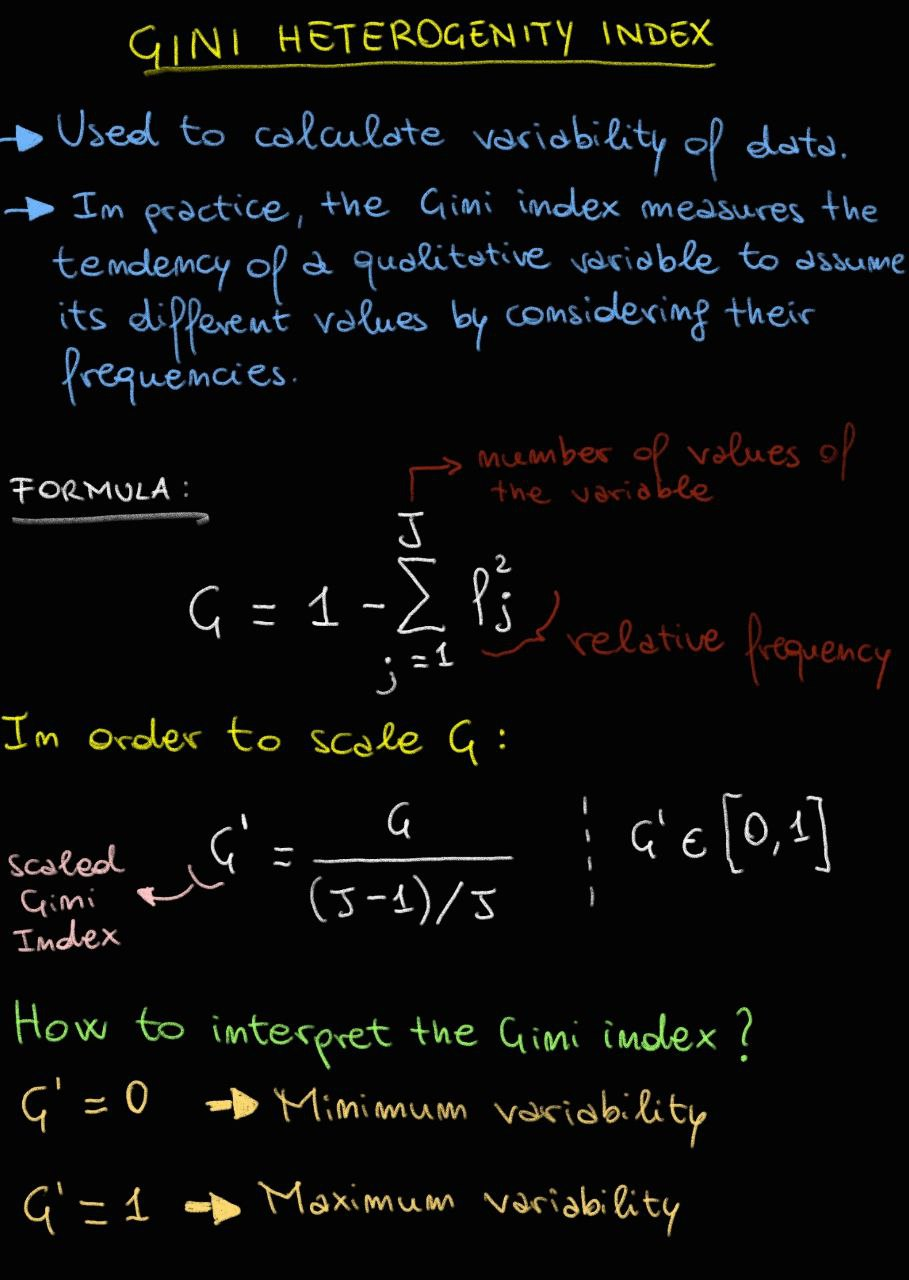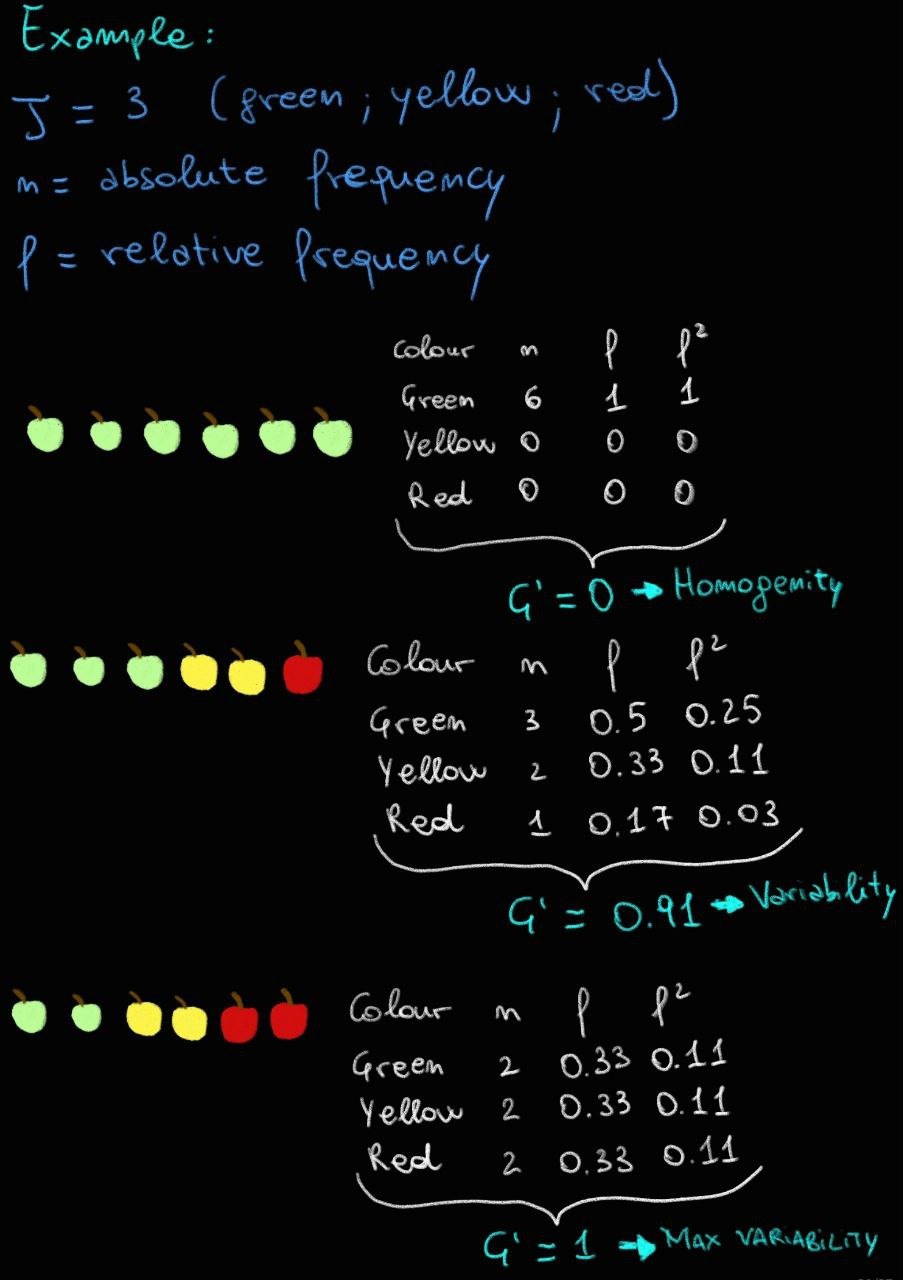I must be honest: summarizing our data using position and variability indices is not sufficient to answer questions like the one posed by our boss: "Can product X increase tomato berry production?" In fact, the observed production values could be influenced by various factors such as favorable or unfavorable weather events, soil conditions, or simply chance. To determine if the observed values and the conclusions drawn from them are statistically significant, an additional method beyond simple data summarization with indices is necessary. This is where hypothesis testing comes into play, but discussing it now would be quite lengthy. I prefer to address this topic in a dedicated article.

In any case, it is crucial to know that the choice of position and variability indices depends on the type of variable being analyzed, the presence or absence of outliers, and the distribution of the data. Regarding the data distribution, it is necessary to delve deeper into the topic in a dedicated article.

I want to remind you that the articles I write are the result of my personal study, which I pursue out of passion and in my free time. The purpose is to share what I learn and to be correct when necessary, so that we can all learn from these resources. I invite you to comment below for any doubts, clarifications, or corrections.

Thank you for reading, and see you soon.

P.S. Below you will find R and Python code for calculating the indices described in the article.

In R:

``````### Create a data frame with all our data:
# Data:
Samples <- 1:40
Trd <- rep(c("Treated"),times=c(20))
Ctrl <- rep(c("Control"),times=c(20))
Condition <- c(Trd, Ctrl)
Number_of_berries_Trd <- round(runif(n=20, min=1, max=20), 0)
Number_of_berries_Ctrl <- round(runif(n=20, min=1, max=10), 0)
Number_of_berries <- c(Number_of_berries_Trd, Number_of_berries_Ctrl)

# Build the dataframe:
df <- data.frame(Samples,
Condition,
Number_of_berries,
row.names = NULL
)
# save the dataframe in a .tsv file
data.table::fwrite(df, "dataframe.tsv", sep = "\t", quote = FALSE)

### Position Index ###

## Mean ----
# Average berries production in Treated plants:
# Select Rows by Condition value
only_Trd <- df[df\$Condition == 'Treated',]
# Calculate mean:
mean_berries_production_in_Trd <- round(mean(only_Trd\$Number_of_berries))
print(mean_berries_production_in_Trd)

# Average berries production in Control plants:
# Select Rows by Condition value
only_Ctrl <- df[df\$Condition == 'Control',]
# Calculate mean:
mean_berries_production_in_Ctrl <- round(mean(only_Ctrl\$Number_of_berries))
print(mean_berries_production_in_Ctrl)

## Median ----
# Median berries production in Treated plants:
# Select Rows by Condition value
only_Trd <- df[df\$Condition == 'Treated',]
# Calculate median:
median_berries_production_in_Trd <- median(only_Trd\$Number_of_berries)
print(median_berries_production_in_Trd)

# Median berries production in Control plants:
# Select Rows by Condition value
only_Ctrl <- df[df\$Condition == 'Control',]
# Calculate median:
median_berries_production_in_Ctrl <- median(only_Ctrl\$Number_of_berries)
print(median_berries_production_in_Ctrl)

## Mode ----
# mode berries production in Treated plants:
# Select Rows by Condition value
only_Trd <- df[df\$Condition == 'Treated',]
# Create a dedicated function for the mode:
get_mode <- function(x) {
u <- unique(x)
tab <- tabulate(match(x, u))
u[tab == max(tab)]
}
# Calculate mode:
mode_berries_production_in_Trd <- get_mode(only_Trd\$Number_of_berries)
print(mode_berries_production_in_Trd)

# mode berries production in Control plants:
# Select Rows by Condition value
only_Ctrl <- df[df\$Condition == 'Control',]
# Create a dedicated function for the mode:
get_mode <- function(x) {
u <- unique(x)
tab <- tabulate(match(x, u))
u[tab == max(tab)]
}
# Calculate mode:
mode_berries_production_in_Ctrl <- get_mode(only_Ctrl\$Number_of_berries)
print(mode_berries_production_in_Ctrl)

## Min and Max ----
# Min and Max berries production in Treated plants:
# Select Rows by Condition value
only_Trd <- df[df\$Condition == 'Treated',]
# Calculate min:
min_berries_production_in_Trd <- min(only_Trd\$Number_of_berries)
print(min_berries_production_in_Trd)
# Calculate max:
max_berries_production_in_Trd <- max(only_Trd\$Number_of_berries)
print(max_berries_production_in_Trd)

# Min and Max berries production in Control plants:
# Select Rows by Condition value
only_Ctrl <- df[df\$Condition == 'Control',]
# Calculate min:
min_berries_production_in_Ctrl <- min(only_Ctrl\$Number_of_berries)
print(min_berries_production_in_Ctrl)
# Calculate max:
max_berries_production_in_Ctrl <- max(only_Ctrl\$Number_of_berries)
print(max_berries_production_in_Ctrl)

## Quantile ----
# Quantile berries production in Treated plants:
# Select Rows by Condition value
only_Trd <- df[df\$Condition == 'Treated',]
# Calculate quantile:
quantile_in_Trd <- quantile(only_Trd\$Number_of_berries, probs = c(0,0.25,0.5,0.75,1))
print(quantile_in_Trd)

# Quantile berries production in Control plants:
# Select Rows by Condition value
only_Ctrl <- df[df\$Condition == 'Control',]
# Calculate quantile:
quantile_in_Ctrl <- quantile(only_Trd\$Number_of_berries, probs = c(0,0.25,0.5,0.75,1))
print(quantile_in_Ctrl)

### Variability Index ###

## Range ----
# range of berries production in Treated plants:
# Select Rows by Condition value
only_Trd <- df[df\$Condition == 'Treated',]
# Calculate range:
range_in_Trd <- range(only_Trd\$Number_of_berries)
print(range_in_Trd)

# range berries production in Control plants:
# Select Rows by Condition value
only_Ctrl <- df[df\$Condition == 'Control',]
# Calculate range:
range_in_Ctrl <- range(only_Ctrl\$Number_of_berries)
print(range_in_Ctrl)

## Interquartile range ----
# Interquartile range of berries production in Treated plants:
# Select Rows by Condition value
only_Trd <- df[df\$Condition == 'Treated',]
# Calculate Interquartile range:
Interquartile_range_in_Trd <- IQR(only_Trd\$Number_of_berries)
print(Interquartile_range_in_Trd)

# Interquartile range berries production in Control plants:
# Select Rows by Condition value
only_Ctrl <- df[df\$Condition == 'Control',]
# Calculate Interquartile range:
Interquartile_range_in_Ctrl <- IQR(only_Ctrl\$Number_of_berries)
print(Interquartile_range_in_Ctrl)

## Variance ----
# Variance of berries production in Treated plants:
# Select Rows by Condition value
only_Trd <- df[df\$Condition == 'Treated',]
# Calculate Variance:
Variance_in_Trd <- IQR(only_Trd\$Number_of_berries)
print(Variance_range_in_Trd)

# Variance berries production in Control plants:
# Select Rows by Condition value
only_Ctrl <- df[df\$Condition == 'Control',]
# Calculate Variance:
Variance_in_Ctrl <- IQR(only_Ctrl\$Number_of_berries)
print(Variance_in_Ctrl)

## Standard Deviation ----
# Standard Deviation of berries production in Treated plants:
# Select Rows by Condition value
only_Trd <- df[df\$Condition == 'Treated',]
# Calculate Standard Deviation:
Standard_Deviation_in_Trd <- sd(only_Trd\$Number_of_berries)
print(Standard_Deviation_in_Trd)

# Standard Deviation berries production in Control plants:
# Select Rows by Condition value
only_Ctrl <- df[df\$Condition == 'Control',]
# Calculate Standard Deviation:
Standard_Deviation_in_Ctrl <- sd(only_Ctrl\$Number_of_berries)
print(Standard_Deviation_in_Ctrl)

## Coefficient of Variation ----
# Coefficient of Variation of berries production in Treated plants:
# Select Rows by Condition value
only_Trd <- df[df\$Condition == 'Treated',]
# Calculate mean and sd
mean_berries_production_in_Trd <- mean(only_Trd\$Number_of_berries)
mean_berries_production_in_Trd <- sd(only_Trd\$Number_of_berries)
# Calculate Coefficient of Variation:
Coefficient_of_Variation_in_Trd <- sd(only_Trd\$Number_of_berries) / mean(only_Trd\$Number_of_berries)
print(Standard_Deviation_in_Trd)

# Coefficient of Variation berries production in Control plants:
# Select Rows by Condition value
only_Ctrl <- df[df\$Condition == 'Control',]
# Calculate mean and sd
mean_berries_production_in_Ctrl <- mean(only_Ctrl\$Number_of_berries)
mean_berries_production_in_Ctrl <- sd(only_Ctrl\$Number_of_berries)
# Calculate Coefficient of Variation:
Coefficient_of_Variation_in_Ctrl <- sd(only_Ctrl\$Number_of_berries) / mean(only_Ctrl\$Number_of_berries)
print(Coefficient_of_Variation_in_Ctrl)

## Index of heterogeneity of Gini ----
# Index of heterogeneity of Gini of berries production in Treated plants:
# Create a function to calculate normalized Gini Index of heterogeneity:
get_geni_index_normalized <- function(x){
# absolute frequency
af = table(x)
# relative frequency
rf = af/length(x)
# squared relative frequency
rf2 = rf^2
# number of values
J = length(table(x))
# gini index formula
gini = 1-sum(rf2)
# Normalized
gini_norm = gini/((J-1)/J)
}
``````

In Python:

``````import pandas as
import numpy as np
from scipy import stats

# Create a data frame with all our data
Samples = np.arange(1, 41)
Trd = np.repeat("Treated", 20)
Ctrl = np.repeat("Control", 20)
Condition = np.concatenate((Trd, Ctrl))
Number_of_berries_Trd = np.round(np.random.uniform(low=1, high=20, size=20))
Number_of_berries_Ctrl = np.round(np.random.uniform(low=1, high=10, size=20))
Number_of_berries = np.concatenate((Number_of_berries_Trd, Number_of_berries_Ctrl))

# Build the dataframe
df = pd.DataFrame({"Samples": Samples, "Condition": Condition, "Number_of_berries": Number_of_berries})

# Save the dataframe in a .tsv file
df.to_csv("dataframe.tsv", sep="\t", index=False)

# Position Index

## Mean
# Average berries production in Treated plants
only_Trd = df[df["Condition"] == "Treated"]
mean_berries_production_in_Trd = round(only_Trd["Number_of_berries"].mean())
print(mean_berries_production_in_Trd)

# Average berries production in Control plants
only_Ctrl = df[df["Condition"] == "Control"]
mean_berries_production_in_Ctrl = round(only_Ctrl["Number_of_berries"].mean())
print(mean_berries_production_in_Ctrl)

## Median
# Median berries production in Treated plants
median_berries_production_in_Trd = only_Trd["Number_of_berries"].median()
print(median_berries_production_in_Trd)

# Median berries production in Control plants
median_berries_production_in_Ctrl = only_Ctrl["Number_of_berries"].median()
print(median_berries_production_in_Ctrl)

## Mode
# Mode berries production in Treated plants
mode_berries_production_in_Trd = stats.mode(only_Trd["Number_of_berries"])
print(mode_berries_production_in_Trd)

# Mode berries production in Control plants
mode_berries_production_in_Ctrl = stats.mode(only_Ctrl["Number_of_berries"])
print(mode_berries_production_in_Ctrl)

## Min and Max
# Min and Max berries production in Treated plants
min_berries_production_in_Trd = only_Trd["Number_of_berries"].min()
print(min_berries_production_in_Trd)
max_berries_production_in_Trd = only_Trd["Number_of_berries"].max()
print(max_berries_production_in_Trd)

# Min and Max berries production in Control plants
min_berries_production_in_Ctrl = only_Ctrl["Number_of_berries"].min()
print(min_berries_production_in_Ctrl)
max_berries_production_in_Ctrl = only_Ctrl["Number_of_berries"].max()
print(max_berries_production_in_Ctrl)

## Quantile
# Quantile berries production in Treated plants
quantile_in_Trd = np.quantile(only_Trd["Number_of_berries"], [0, 0.25, 0.5, 0.75, 1])
print(quantile_in_Trd)

# Quantile berries production in Control plants
quantile_in_Ctrl = np.quantile(only_Ctrl["Number_of_berries"], [0, 0.25, 0.5, 0.75, 1])
print(quantile_in_Ctrl)

# Variability Index

# Range
only_Trd = df[df['Condition'] == 'Treated']
range_in_Trd = np.ptp(only_Trd['Number_of_berries'])
print(range_in_Trd)

only_Ctrl = df[df['Condition'] == 'Control']
range_in_Ctrl = np.ptp(only_Ctrl['Number_of_berries'])
print(range_in_Ctrl)

# Interquartile range
only_Trd = df[df['Condition'] == 'Treated']
Interquartile_range_in_Trd = np.percentile(only_Trd['Number_of_berries'], 75) - np.percentile(only_Trd['Number_of_berries'], 25)
print(Interquartile_range_in_Trd)

only_Ctrl = df[df['Condition'] == 'Control']
Interquartile_range_in_Ctrl = np.percentile(only_Ctrl['Number_of_berries'], 75) - np.percentile(only_Ctrl['Number_of_berries'], 25)
print(Interquartile_range_in_Ctrl)

# Variance
only_Trd = df[df['Condition'] == 'Treated']
Variance_in_Trd = np.var(only_Trd['Number_of_berries'])
print(Variance_in_Trd)

only_Ctrl = df[df['Condition'] == 'Control']
Variance_in_Ctrl = np.var(only_Ctrl['Number_of_berries'])
print(Variance_in_Ctrl)

# Standard Deviation
only_Trd = df[df['Condition'] == 'Treated']
Standard_Deviation_in_Trd = np.std(only_Trd['Number_of_berries'])
print(Standard_Deviation_in_Trd)

only_Ctrl = df[df['Condition'] == 'Control']
Standard_Deviation_in_Ctrl = np.std(only_Ctrl['Number_of_berries'])
print(Standard_Deviation_in_Ctrl)

# Coefficient of Variation
only_Trd = df[df['Condition'] == 'Treated']
mean_berries_production_in_Trd = np.mean(only_Trd['Number_of_berries'])
sd_berries_production_in_Trd = np.std(only_Trd['Number_of_berries'])
Coefficient_of_Variation_in_Trd = sd_berries_production_in_Trd / mean_berries_production_in_Trd
print(Coefficient_of_Variation_in_Trd)

only_Ctrl = df[df['Condition'] == 'Control']
mean_berries_production_in_Ctrl = np.mean(only_Ctrl['Number_of_berries'])
sd_berries_production_in_Ctrl = np.std(only_Ctrl['Number_of_berries'])
Coefficient_of_Variation_in_Ctrl = sd_berries_production_in_Ctrl / mean_berries_production_in_Ctrl
print(Coefficient_of_Variation_in_Ctrl)

# Index of heterogeneity of Gini
def get_gini_index_normalized(x):
af = np.histogram(x, bins=len(np.unique(x)))
rf = af / len(x)
rf2 = rf**2
J = len(np.unique(x))
gini = 1 - np.sum(rf2)
gini_norm = gini / ((J - 1) / J)
return gini_norm

only_Trd = df[df['Condition'] == 'Treated']
gini_index_normalized_in_Trd = get_gini_index_normalized(only_Trd['Number_of_berries'])
print(gini_index_normalized_in_Trd)
``````